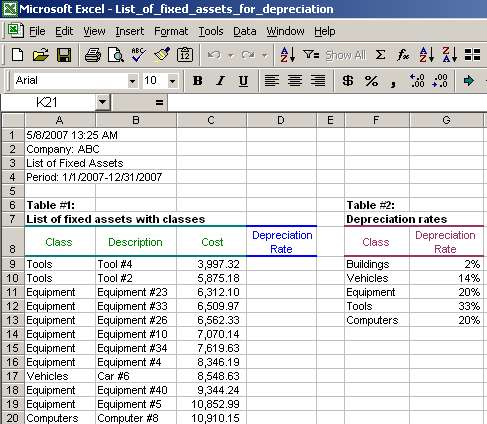# Vlookup Function, Vlookup Syntax, Vlookup Examples

## This Excel lecture explains VLOOKUP function and VLOOKUP syntax in Excel. One VLOOKUP example is provided to illustrate the benefits of using this function. Read this VLOOKUP tutorial to see that VLOOKUP is a very powerful function in Excel.

The company name and numbers in this lecture are not real and no association with a real company with a similar name, if such exists, should be made.

### 1) Vlookup function and syntax

Example of VLOOKUP syntax and list of its arguments are presented below. There are white spaces presented in the example; in some earlier Excel versions such white spaces will cause an error message and in some later Excel versions, white spaces are treated without problems. So, if your Excel version gives you an error, just delete all white spaces:

= VLOOKUP ( lookup_value, table_array, col_index_num, range_lookup )

Note that VLOOKUP has 4 arguments. Let's review the function arguments in more detail:

• lookup_value means the value to be found in the first column of the table_array. This is a key by which two tables (the table in which lookup_value is stored and the table_array in which you want to lookup a related value) are linked together;
• table_array means the table of information in which data is looked up. Usually, table_array is separate from the table in which the lookup_value is stored;
• col_index_num means the column number in table_array from which the matching value must be returned. A col_index_num of 1 returns a value in the first column in table_array; a col_index_num of 2 returns a value in the second column in table_array, and so on. In other words, VLOOKUP will pull a related value from the column equal to col_index_num counted from the first column in table_array.
• range_lookup means a logical value that specifies whether you want VLOOKUP to find an exact match or an approximate match. If TRUE or omitted, an approximate match is returned. In other words, if an exact match is not found, the next largest value that is less than lookup_value is returned. If FALSE, VLOOKUP will find an exact match. If one is not found, the error value #N/A is returned. FALSE usually has the most use in my work because of the need to lookup an exact match and if there is no such match, to obtain the error value #N/A.

We will take a look at an example to see how all above is tied together.

### 2) Vlookup example: Find a match for depreciation rates for fixed asset classes

We have a list of fixed assets with fixed asset classes (Table #1, cells A8-D179 in the list of fixed assets with classes). We also have a table showing depreciation rates for each fixed assets class (Table #2, cells F8-G13). What we need to do is assign each fixed asset its depreciation rate based on the fixed asset class (see the list of fixed assets with classes):Column D in Table #1 is where we want the depreciation rates to be entered from Table #2.

Page 1 of 3

Next